Reach Us+44-1474-556909
Stability of Convergence Theorems of the Noor Iteration Method for an Enumerable Class of Continuous Hemi Contractive Mapping in Banach Spaces | OMICS International
Journal of Applied & Computational Mathematics
All submissions of the EM system will be redirected to Online Manuscript Submission System. Authors are requested to submit articles directly to Online Manuscript Submission System of respective journal.

# Stability of Convergence Theorems of the Noor Iteration Method for an Enumerable Class of Continuous Hemi Contractive Mapping in Banach Spaces

Akanksha Sharma1*, Kalpana Saxena2 and Namrata Tripathi1

*Corresponding Author:
Akanksha Sharma
Department of Mathematics, Technocrats Institute of Technology
Tel: 07552751679
E-mail: [email protected]

Received June 25, 2015; Accepted July 27, 2015; Published July 30, 2015

Citation: Sharma A, Saxena K, Tripathi N (2015) Stability of Convergence Theorems of the Noor Iteration Method for an Enumerable Class of Continuous Hemi Contractive Mapping in Banach Spaces. J Appl Computat Math 4: 239. doi:10.4172/2168-9679.1000239

Copyright: © 2015 Sharma A, et al. This is an open-access article distributed under the terms of the Creative Commons Attribution License, which permits unrestricted use, distribution, and reproduction in any medium, provided the original author and source are credited.

Visit for more related articles at Journal of Applied & Computational Mathematics

#### Abstract

The purpose of this is to study the Noor iteration process for the sequence {xn} converges to a common fix point for enumerable class of continuous hemi contractive mapping in Banach spaces.

#### Keywords

Stability; Noor iterations; Hemicontractive mapping; Convergence theorem; Continuous pseudocontractive mapping

2000 Mathematics Subject Classification: 47J25, 47H10, 54H25

#### Introduction

Let E be a real Banach space and let J denote the normalized duality mapping from E to E* defined by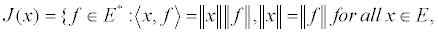Where E* denotes the dual space of E and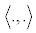denotes the generalization duality pair.

It is well known that if E* is strictly convex then J is single–valued. In the sequel, we shall denote the single–valued duality mapping by j. Let k be a nonempty closed convex subset of Banach space E and T : K → K be a self-mapping of K.

Definition 3.1: (i) A mapping T with domain D(T) and range R(T) in a Banach space is called pseudocontrative mapping, if for all x, y∈ D(T), there exists j(x − y)∈ J (x − y) such that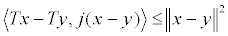(1)

(ii) A mapping T with domain D(T) and range R(T) in E is called a hemicontractive mapping if F (T) ≠∅ and for all x∈D(T)x* ∈F(T) such that,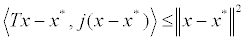(iii) A mapping T : K → K is called L-Lipschitizan there exists L>0 such that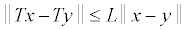For all x, y∈K

Definition 3.2: If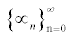and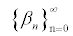are sequences of real numbers in [0, 1] . For arbitrary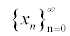be a Noor iteration defined by,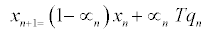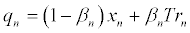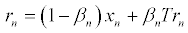Lemma 3.3: Let E be a real uniformly convex Banach space , K is nonempty closed convex subset of E and T a continuous pseudocontractive mapping of K, then I-T is demiclosed at zero, that is, for all sequences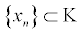with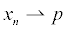and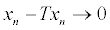it follows that p = Tp

Lemma 3.4: Let δ be a number satisfying 0 ≤δ <1 and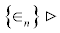a positive sequence satisfying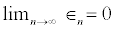[4,5]. Then, for any positive sequence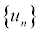satisfying: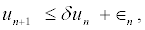It follows that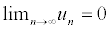.

#### Results

Theorem 4.1: Let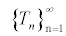be defined as above and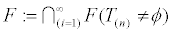i n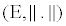and let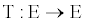be a Banach space, T : E→E a self-map of E with a fixed point p, satisfying the contractive condition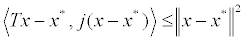for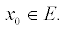Let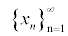is converge to p and defined by the iteration (3.2) where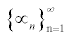is a real sequence in (0, 1) and define as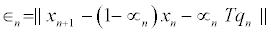Then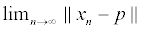exists for all p∈ F;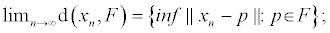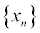converges strongly to a common fixed point of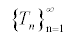if and only if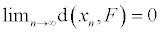Proof: Let p∈ F and n ≥1 by 3.1 we choose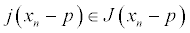such that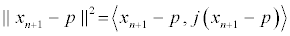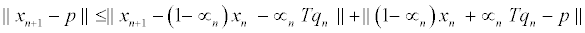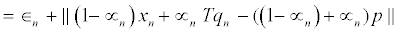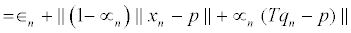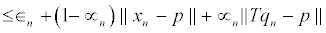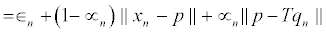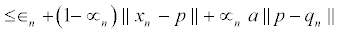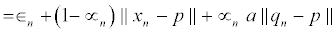(1)

For the estimate of in (1) we get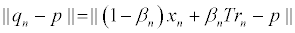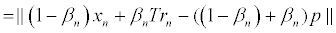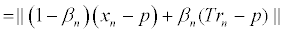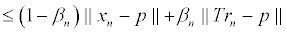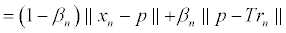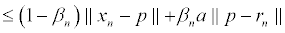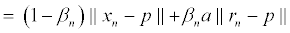(2)

Substituting (2) into (1) gives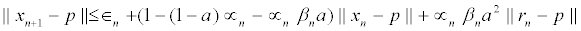(3)

For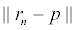in (3) we have,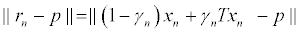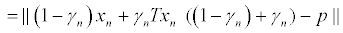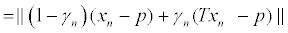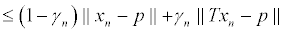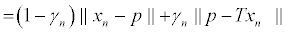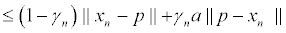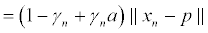(4)

Substituting (4) into (3) and using lemma 3.3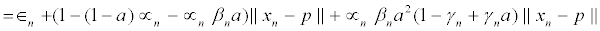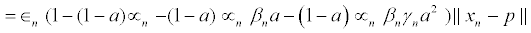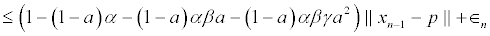Observe that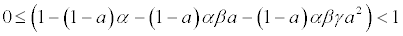(5)

Therefore, taking the limit as n→∞ of both sides of the inequality (5) and using lemma 1.6 we get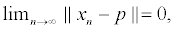That is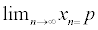By theorem 3.2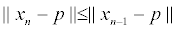Taking infimum over all p∈F,we have,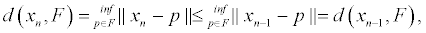Thus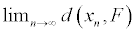exist we finally prove (iii) suppose that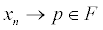Ffrom (ii) and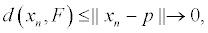We have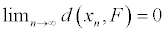for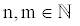and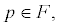, it follows

From (1.3) that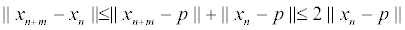Consequently,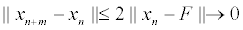Therefore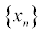is a Cauchy sequence. Suppose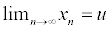for some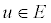. Then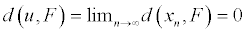Since F is closed set, u∈F

So, Noor iteration process is T –stable.

#### Conclusion

Thus, the stability of Noor iteration considerable for finding fixed point for enumerable class of continuous hemi contractive mapping in Banach spaces.

#### References

Select your language of interest to view the total content in your interested language

### Article Usage

• Total views: 12071
• [From(publication date):
July-2015 - Jul 23, 2019]
• Breakdown by view type
• HTML page views : 8285Can't read the image? click here to refresh